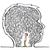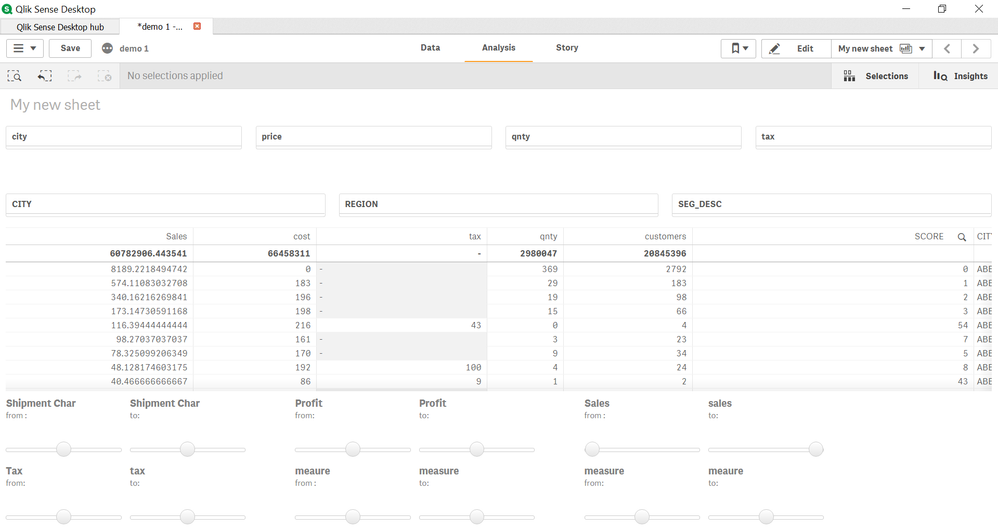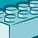Announcements
Applications are open for the 2024 Qlik Luminary Program. Apply by December 15 here.
cancel
Showing results for
Did you mean:Contributor III

## Range filter

isn't there any other way to just give simple range filters where user has option of   > and < operator or can have  between clause, in simple words just a ranged filter.

This functionality is so much easier in tableau and power hence in qlik sense i haven't came across one fully functional solution.

my solution :

vMax =max(sales)

vMin=max(sales)

then use above variables in sales measure like this :

= if((sum(Sales) >= \$(vMin) AND sum(Sales) <= \$(vMax)),sum(TotalCalls))

which gives me following output in screenshot no 5  filtering the data but leaving blank in sales column where condition not met:

Labels (79)

• ### Script

1 Solution

Accepted SolutionsMVP

You can suppress those rows by going into the following properties: Add-ons -> Data handling -> Uncheck 'Include zero values'.

For the total, you can just change your expression to

``````Sum(Aggr(
If((Sum(Sales) >= \$(vMin) and Sum(Sales) <= \$(vMax)), Sum(TotalCalls))
, YourDimensionsFromTheChart))``````

5 RepliesMVP

You can suppress those rows by going into the following properties: Add-ons -> Data handling -> Uncheck 'Include zero values'.

For the total, you can just change your expression to

``````Sum(Aggr(
If((Sum(Sales) >= \$(vMin) and Sum(Sales) <= \$(vMax)), Sum(TotalCalls))
, YourDimensionsFromTheChart))``````Contributor III
Author

my dimension has millions of distinct values which is actually customer no , can you guide in this matter secondly how can i apply the selection made from my range filter to other sheets.

note : i have made multiple range filters.MVP

I am not sure I understand the issue? "my dimension has millions of distinct values which is actually customer no" What does that have to do with the solution provided.

For the second condition... what exactly are you trying to do. Again, I am not really sure about the selections you are looking to have dashboard wide?Contributor III
Author

hi,

below is what i'm trying to do, creating normal filters and range filters for columns having alot of distinct values .

Requirement : using multiple filters lock the customers on certain criteria eg :

customer having sales > 5000  and qnty < 50 and profit  between 200 and 1000

then show count(Distinct customers) with other dimensions.

after this sheet will be individual customer level sheet showing each customer who fulfilled above eg. criteria with some other kpis.

Issues :

1)filters should be applied per customer

2)totals should also change

3)dependencies between normal filter and range filters and with themselves too.

4) selection done in ranged filters don't get refreshed plus they don't show up in top selected tab too which makes it difficult to under which filter is applied which is not.

logic used in columns :

created two variables min and max then applied below expression in table columns :

= if((sum(Tax) >= \$(vMinTax) AND sum(Tax) <= \$(vMaxTax)),
if(isnull(sum(Tax)),null(),sum(Tax))
,null()
)Contributor

Hi, I have multiple sheets with different KPIs and tables, how can I make the range filter selection apply to all of them? Do I need to specify such an expression for every single chart and custom objects I have used?Tags
Community Browser